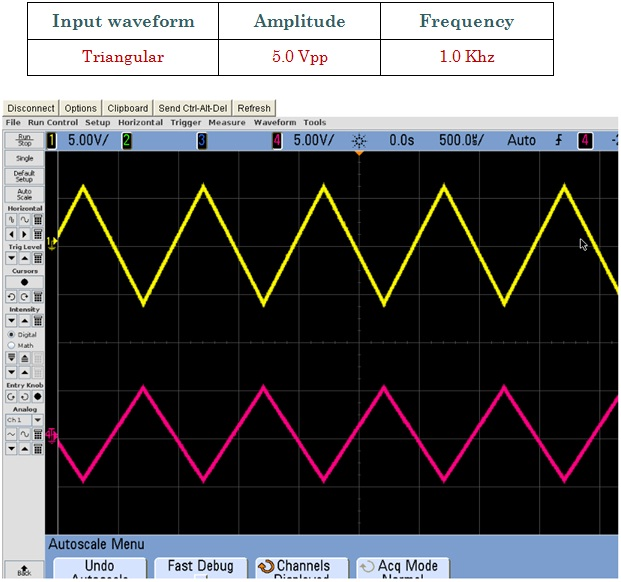Inverting Amplifier

Calculations & Observations

There are two very important rules to remember about Inverting Amplifiers or any operational amplifier for that matter and these are.

1. No Current Flows into the Input Terminals
2. The Differential Input Voltage is Zero as V1 = V2 = 0 (Virtual Earth)
Then by using these two rules we can derive the equation for calculating the closed-loop gain of an inverting amplifier, using first principles.
Current (i) flow through the resistor network as shown.Fig.1 Inverting Amplifier circuitThe negative sign in the equation indicates an inversion of the output signal with respect to the input as it is 180° out of phase. This is due to the feedback being negative in value. The equation for the output voltage Vout also shows that the circuit is linear in nature for a fixed amplifier gain as, Vout = Vin x Gain. This property can be very useful for converting a smaller sensor signal to a much larger voltage.Fig.2 Shows Input Signal (Red) and Output Signal (Yellow)Fig.3 Shows Input Signal (Red) and Output Signal (Yellow)